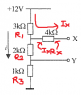# Voltage divider question

• TsAmE

## Homework Statement

Calculate the current i and the voltage appearing at X and Y

None.

## The Attempt at a Solution

I found I to be 0.002A and Vy = 2V, but I am having trouble with Vx

Attempt:

I tried getting the current that goes through the 3k and 4k resistors:

Ix = V / (R1 + Rx)
= 12 / ((3 + 4) x 10^3)
= 1.71 x 10^-3 A

I then calculated the voltage on the first line after the 3k resistor:

Vx' = V(R2 + R3) / (R1 + R2 + R3)
= (12(2 + 1) x 10^3) / (3 + 2 + 1) x 10^3
= 6V

I then subtracted the Vdrop across the 4k resistor from Vx' to get the voltage at X:

Vx = Vx' - IxRx
= 6 - (1.71 x 10^-3)(4 x 10^3)
= -0.84V

but the correct answer was Vx = 6V. How can this be possible? Why isn't there a voltage drop across the 4k resistor?

#### Attachments

•Voltage divider 1.png
3.1 KB · Views: 388